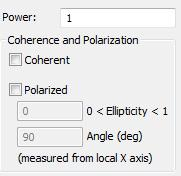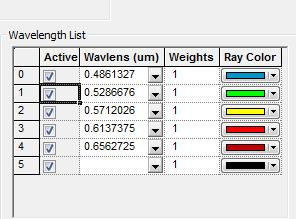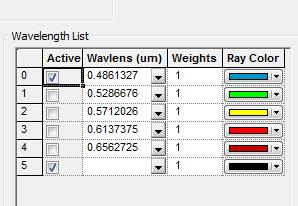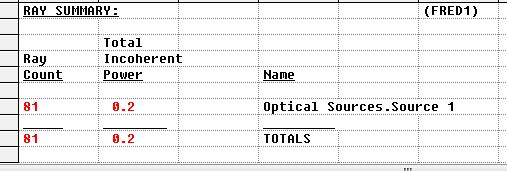# Source Wavelength List and Power

When using a source whose spectral content is defined using the Wavelength List option, each of the specified wavelengths contributes to some fraction of the total specified source power.  If a wavelength is toggled as Active, then its contribution to the total source power will be included in the rayset.  If a wavelength's Active flag is not toggled, then it's contribution to the total source power will not be included in the generated rayset.  Philosophically, FRED considers the specified source Power value to be an intrinsic source property rather than a normalized quantity with respect to the subset of Active wavelengths in the wavelength list.

In the example below, the intrinsic source power is specified as 1.Five discrete wavelength values are specified, with the total power being equally distributed among them as indicated by the uniform wavelength Weights.However, if we toggle the Active state of any of the wavelengths, the power in each remaining active wavelength is not re-normalized to equal the specified total source power value of 1.0 (to renormalize, you must either delete the unused wavelengths or adjust the Power setting).  Rather, the power contributions from the inactive wavelengths is simply removed from the generated rayset.In this example, the equally weighted wavelengths each contribute 0.2 units of power and give a sum total power of 1.0 when all wavelengths are Active.  With only one wavelength active, the generated rayset contains only the active wavelength's contribution to the source's intrinsic power.  In this case, the rayset contains only 0.2 units of power.